Algebra 1 : How to write expressions and equations

Example Questions

Example Question #141 : How To Write Expressions And Equations

Which of the following English-language sentences can be written as the equation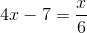?

Seven subtracted from the product of a number and four yields the quotient of the number and six.

The product of a number and four subtracted from seven yields the quotient of the number and six.

The product of a number and four subtracted from seven yields the quotient of six and the number.

None of the other responses yields a correct answer.

Seven subtracted from the product of a number and four yields the quotient of six and the number.

Seven subtracted from the product of a number and four yields the quotient of the number and six.

Explanation:

Letbe the unknown number in question.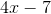is seven subtracted from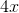, which in turn is the product of four and a number. Therefore, the expression can be written as "seven subtracted from the product of a number and four".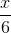can be written as "the quotient of six and the number".

Therefore,can be written as "Seven subtracted from the product of a number and four yields the quotient of the number and six."

Example Question #142 : How To Write Expressions And Equations

Write the expression:  Twice a number less than five.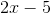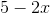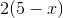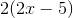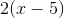Explanation:

Split the sentence into parts.  Let a variable be the unknown number.

Twice a number:Twice a number less than five means that twice a number will be subtracted from five.

The answer is:Example Question #143 : How To Write Expressions And Equations

Write the following equation:  Five times the cube root of three times a number is six.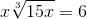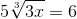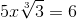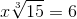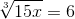Explanation:

Split the sentence into parts:

Three times a number:The cube root of three times a number: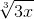Five times the cube root of three times a number: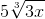Is six: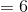Combine the terms.

The correct answer is:Example Question #144 : How To Write Expressions And Equations

Write the expression:  Sixteen less than three times a number.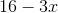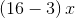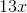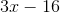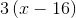Explanation:

Break up the sentence into parts.

Three times a number:Sixteen less than three times a number:The answer is:Example Question #145 : How To Write Expressions And Equations

Write the equation:   Twice the quantity of a number less than sixteen is four.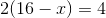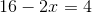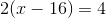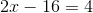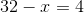Explanation:

Break up the sentence into parts.

A number less than sixteen: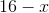Twice the quantity of a number less than sixteen: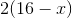Is four: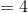The equation is:Example Question #146 : How To Write Expressions And Equations

Write the following expression:  The difference of twice a number and the number squared.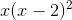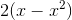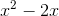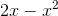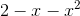Explanation:

Break up the sentence by parts.

Twice a number:The number squared:The difference of twice a number and the number squared:The answer is:Example Question #147 : How To Write Expressions And Equations

Write the following expression:  Three less than a number squared.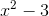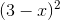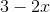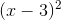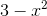Explanation:

Split the question into parts.

A number squared:Three less than a number squared:The answer is:Example Question #148 : How To Write Expressions And Equations

Write the expression:  The sum of twice a number and fifty.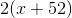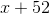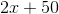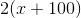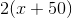Explanation:

Break up the sentence into parts.

Twice a number:The sum of twice a number and fifty:The answer is:Example Question #149 : How To Write Expressions And Equations

Write the equation:  The cube root of half the number is five.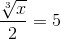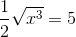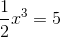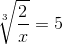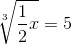Explanation:

Split up the sentence into parts.

Half the number: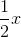The cube root of half the number: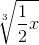Is five: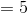Combine the terms to form an equation.

The answer is:Example Question #150 : How To Write Expressions And Equations

Write the expression:  A number squared less than two.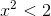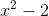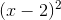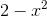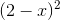Explanation:

Split the sentence into parts.

A number squared:A number squared less than two means that the number squared will be smaller than two.

Do not mix up expressions with equations or inequalities because expressions do not contain an equal sign.

The answer is: